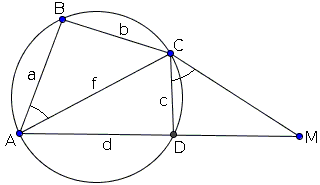# Construction of a Cyclic Quadrilateral

Construct a cyclic quadrilateral, given the lengths of its sides in a prescribed order.

Given $4 numbers$a,b,c,d$which are assumed to be the successive side lengths of a quadrilateral, if one of them is greater than the sum of the remaining three, the construction is rather obviously impossible. There is no quadrilateral whose sides satisfy, say,$a \gt b + c + d.$Such cases aside, the construction is always possible. ConstructionCopyright © 1996-2018 Alexander Bogomolny Construct a cyclic quadrilateral, given the lengths of its sides in a prescribed order. Assume the desired cyclic quadrilateral exists:Draw$CM,$with$M$on the extension of$AD,$so that$\angle DCM = \angle BAC.$This makes triangles$ABC$and$CDM$similar because$\angle ABC = 180^{\circ} - \angle ADC = \angle CDM.$The similarity of the triangles implies a proportion$DM/b = c/a,$so that$DM = bc/a.$Additionally,$CM/f = c/a,$which tells us that$C$is located on the locus of point whose distances to points$A$and$M$are in a given ratio$c/a.$The locus is an Apollonian circle. This information is sufficient to carry out the construction. (In the following$C(O, r)$will denote the circle with center$O$and radius$r.)$Let$d$be the largest of the four given lengths. 1. Draw$AD = d$and extend it to$M$so that$DM = bc/a.$2. Find$C$at the intersection of two circles:$C(D, c)$and the Apollonian circle defined by$AC/CM = c/a.$3.$B$is found at the intersection of two circles$C(A, a)$and$C(C, b).$The circle of Apollonius crosses$AM$at point$K$such that$AK$= (ad + bc)/(a + c).$ $(AM = d + bc/a,$ and this is divided in the ratio $c/a.)$

$K$ is to the left of or on $D$ exactly when $AK \le d$ or, equivalently, $b \le d.$ This condition holds by the choice of $d$ as the largest of the four numbers.

If so, $KD = d - (ad + bc)/(a + c) = (cd - bc)/(a + c).$ This is less than $c:$

$(cd - bc)/(a + c) - c = [c/(a + c)](d - b - a - c) \lt 0.$

Thus we can be sure that in the second step the two circles intersect.

In the third step, we simply get two triangles $ABC$ and $CDM$ with proportional sides, so that the two are similar. In particular, $\angle ABC = \angle CDM$ or, which is the same, $\angle ABC + \angle ADC = 180^{\circ}.$ And so the quadrilateral $ABCD$ is cyclic.

The construction determines the quadrilateral uniquely, however, if we disregard the order of the sides, there are, in principle, 6 different cyclic quadrilaterals with sides $a,$ $b,$ $c,$ and $d.$ All have the same area given by Brahmagupta's formula:

$S = \sqrt{(s - a)(s - b)(s - c)(s - d)},$

where $s$ is the semiperimeter of the quadrilateral: $s = (a + b + c + d)/2.$

All six also share the circumcircle which follows from the formula for the circumradius:

$16R^2 = (ad + bc)(ac + bd)(ab + cd) / [(s - a)(s - b)(s - c)(s - d)].$

### References

1. R. A. Johnson, Advanced Euclidean Geometry (Modern Geometry), Dover, 1960, pp. 82-83### Various Geometric Constructions

• How to Construct Tangents from a Point to a Circle
• How to Construct a Radical Axis
• Constructions Related To An Inaccessible Point
• Inscribing a regular pentagon in a circle - and proving it
• The Many Ways to Construct a Triangle and additional triangle facts
• Easy Construction of Bicentric Quadrilateral
• Easy Construction of Bicentric Quadrilateral II
• Star Construction of Shapes of Constant Width
• Four Construction Problems
• Geometric Construction with the Compass Alone
• Construction of n-gon from the midpoints of its sides
• Short Construction of the Geometric Mean
• Construction of a Polygon from Rotations and their Centers
• Squares Inscribed In a Triangle I
• Circle of Apollonius
• Six Circles with Concurrent Pairwise Radical Axes
• Trisect Segment: 2 Circles, 4 Lines
• Tangent to Circle in Three Steps
• Regular Pentagon Construction by K. Knop
•